# Linear Regression 面试常见考点解析Linear regression 模型在数据科学岗位日常工作中有广泛应用， 同时它也是数据科学岗位面试的常见考点。在资深面试官眼中的数据科学案例分析面试一文中，我们多次提到面试中非常注重求职者对机器学习基础知识点的深刻理解，而 linear regression 就是最重要的基础知识考点之一。在本文中，我们会结合面试例题给大家精炼梳理 linear regression 相关知识，在随后的系列文章中， 我们会逐一讨论与 linear regression 有紧密联系的 logistic regression, softmax regression 等内容。## 1. Linear Regression概念介绍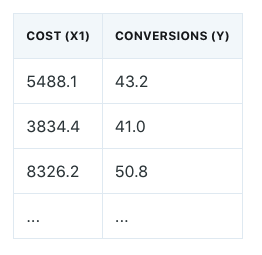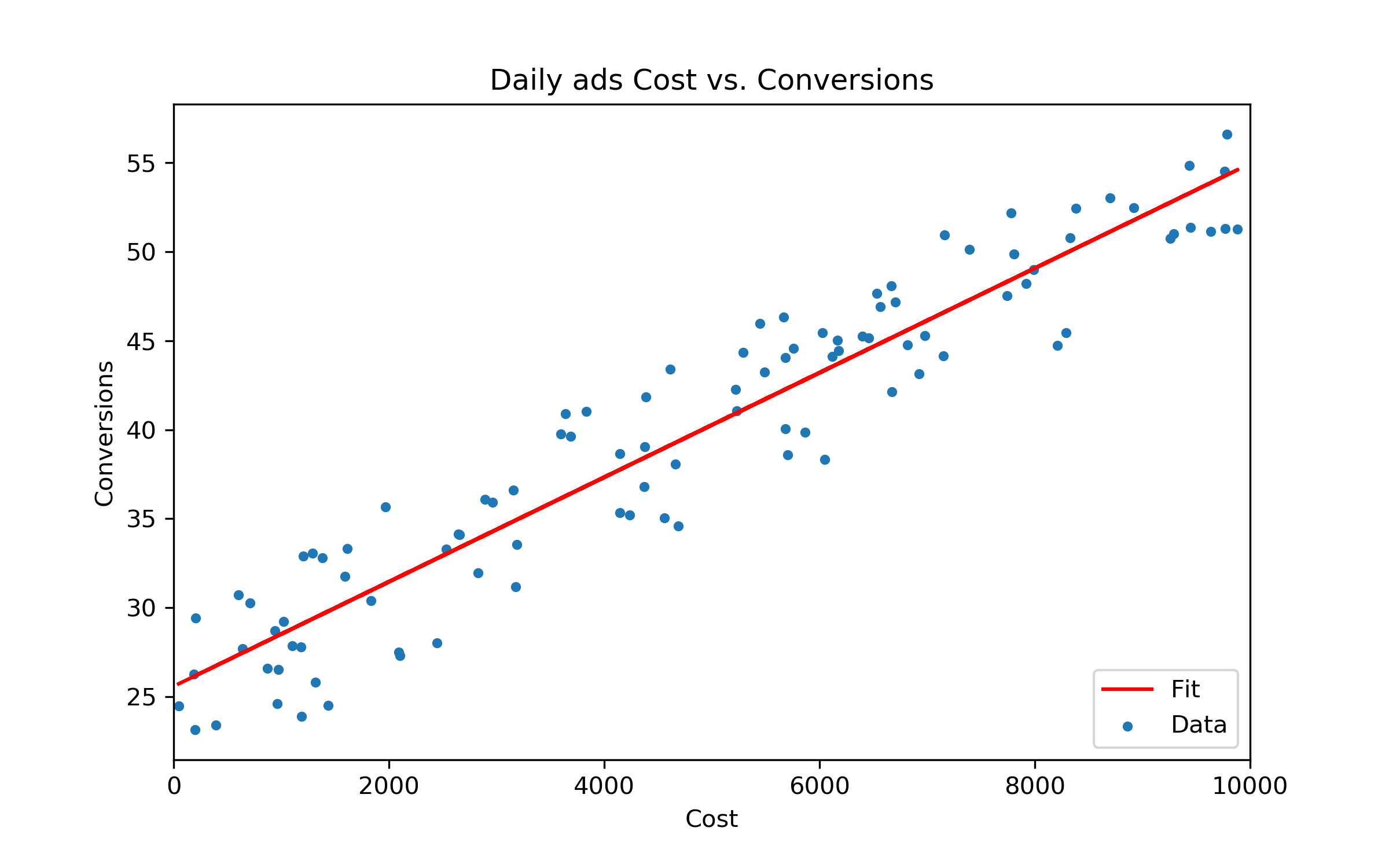在这里先对一些数据符号进行说明：我们用 ${x}_{1}^{\left(i\right)}$ 表示训练数据 (training data) 里第 i 行观测数据中的 cost。这里的上角标i表示第 $i$ 行数据，下角标$1$表示训练数据里的第1个 feature。在上述例子中，我们只有一个 feature ${x}_{1}$，也就是广告 Cost。在现实应用中我们可以有很多 features, 比如广告的类别，广告的点击率等等，在那些情况下， 我们就会有 ${x}_{2}$等等。${y}^{\left(i\right)}$ 表示训练数据中第i行对应的 Conversions。由于我们这里讨论 linear regression, 因此我们在数据建模时就用以下的 hypothesis function 来通过 估计 :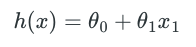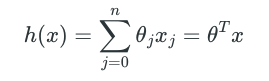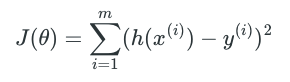Notes 1: 我们求解 linear regression 中参数的时候， 并不一定要用 Ordinary Least Squares 这个 estimator。Ordinary Least Squares 只是 Least Squares estimation 中的一种方法，除此之外我们还可以用 Weighted Least Squares (WLS)，Generalized Least Squares (GLS) 等方法，我们甚至可以抛弃Least Squares, 改用 Least Absolute Deviations (LAD) 来解决 linear regression问题。因此，大家要注意，在我们选用 linear regression作为 model 之后，我们可以选择任意estimator作为 loss function 的定义方法，Ordinary Least Squares不是唯一方法。

## 2. 从概率角度理解OLS Linear Regression

1. The dependent variable $y$ and independent variable $x$ follow the linear form $y={\theta }^{}x+ϵ$, where $ϵ$ represents unpredictable random noise.
2. The error term $ϵ$ is independently and identically distributed. Additionally, the error term $ϵ$ has a population mean of zero and a constant variance, i.e. $E\left(ϵ\right)=0$$Var\left(ϵ\right)={\mathrm{\sigma ^}}^{2}$.
3. (Optional) The error term $ϵ$ follows a normal distribution with mean zero and constant variance ${\mathrm{\sigma ^}}^{2}$.

Note 2: 在assumption 1中我们对error term的unpredictable random noise的定义也同时默认表示there is no correlation between the independent variables $x$ and the error term $ϵ$。在有些资料中，no correlation这一点会被单独列出来。

Note 3: Assumption 3 中对 error term 的 normal distribution assumption 并不是OLS linear regression必需的。只不过 normally distributed error term会使得 OLS 成为 the most efficient linear regression estimator。建议大家在面试时可以直接把这个3个 assumption 都讲出来，在绝大多数情况下面试官是会满意的。 极个别情况下，如果面试官对这个答案提出质疑， 我们可以再进一步讨论第3个 assumption 是 optimal 的。在后文中，我们会默认同时使用这3个 assumptions。

Given the assumption $ϵ\sim N\left(0,{\mathrm{\sigma ^}}^{2}\right)$, we can get its probability density function as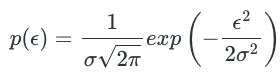It implies that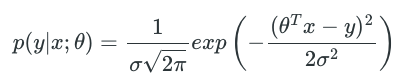Based on the principal of maximum likelihood estimation, we should choose $\theta$ so as to maximize likelihood function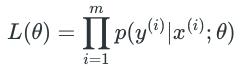In order to simplify our derivations, we maximize its log likelihood as following: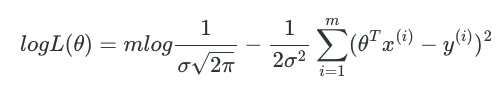As we can see here, maximizing $logL\left(\theta \right)$ is the same as minimizing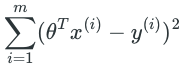## 3. Loss function数值求解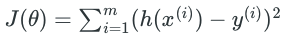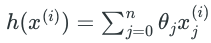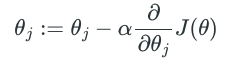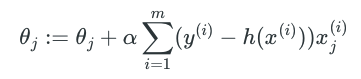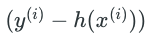## 4. 总结

Linear regression虽然是最简单的机器学习模型，但在数据科学岗位面试中出现的频率并不低，考点主要涉及 model assumptions 的细节以及 loss function 的相关推导和概念理解。本文从统计机器学习角度把相关常见考点进行了梳理汇总， 至于对 Linear regression 参数估计结果的 statistical hypothesis testing 等数理统计的内容，我们会在后续的文章中继续讨论。最后给大家留一个思考题：

Question: 基于本文中提到的 OLS linear regression 的3个基本假设（包含第三个assumption: the error term follows normal distribution), 我们是否可以认为：linear regression assumes the dependent variable $y$ itself follows normal distribution?